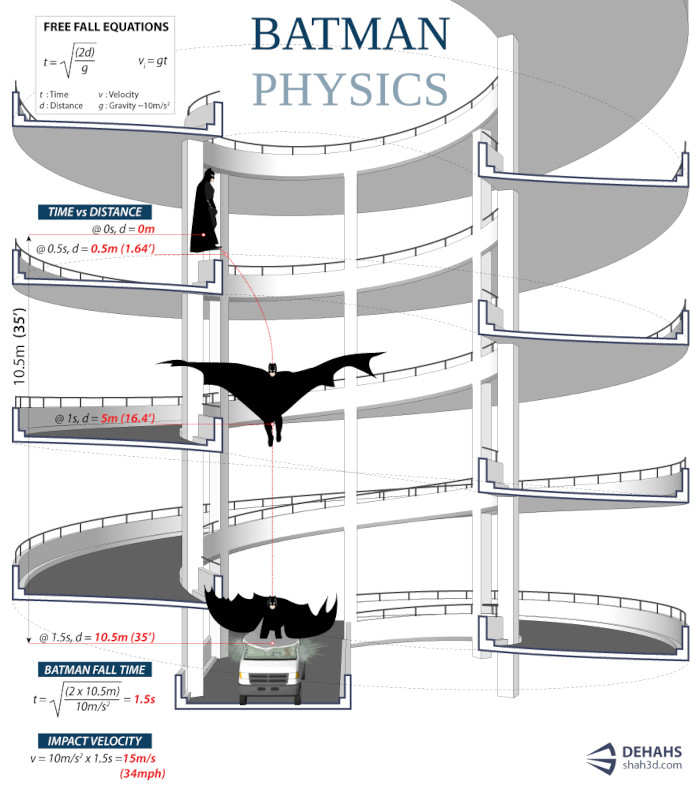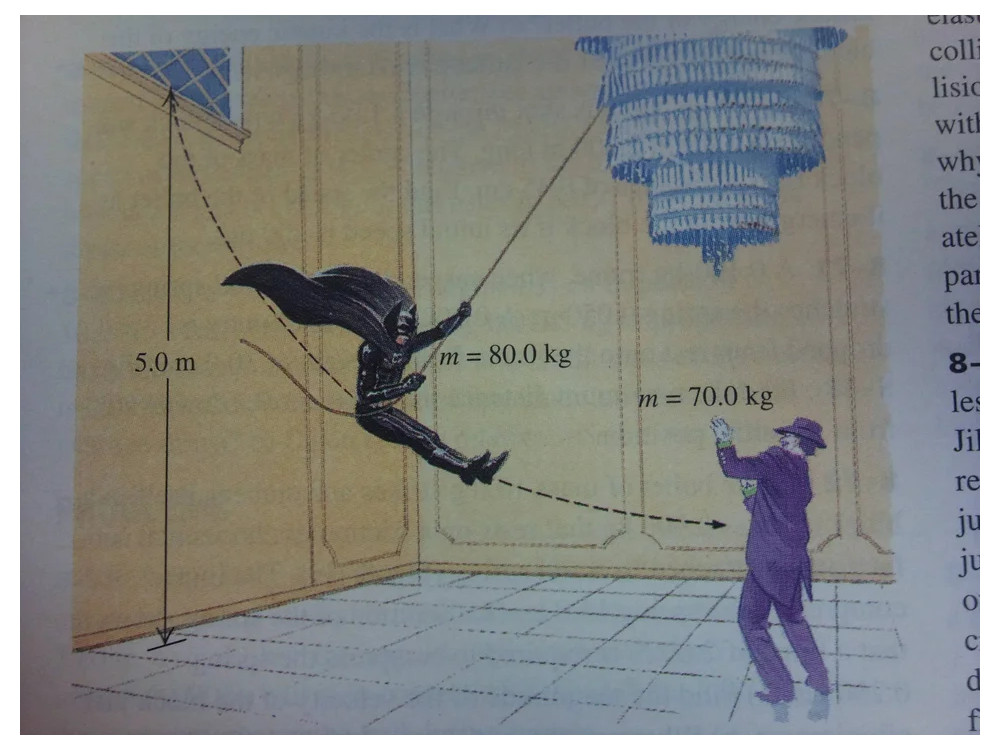### The flying BatmanComics' authors, and more generally those who write about fiction (but not only them), are the modern Scheherazade of One Thousand and One Nights. This comparison is very fitting for Detective Comics #1027 which celebrates at the same time #27, where Bob Kane and Bill Finger's Batman made his debut, and the 1001th issue of the magazine dedicated to the Caped Crusader, so you understand the initial comparison.
I'm not here, though, to write you why Detective Comics's 1001 releases with Batman (if you want to, follow note(1)), but to seize the opportunity and talk about Batman's physics.
Hanging on the batrope
Since his debut in Detective Comics #27 Batman has been immortalized on the cover in an acrobatic pose: hanging on a rope while grabbing a criminal over the rooftops of Gotham. However, Batman's aerial acrobatics are not limited to just hanging from his rope, but sometimes he launches himself from tall buildings or glides using hang gliders or a particular rigid version of his mantle.
If we take the process of the downward fall, this is very well described by the infographic below created by designer Shahed Syed in 2012 on the occasion of the release of the last chapter of Christopher Nolan's Batman's trilogy:The infographic is based, as well written in the text, on the free fall equations, in particular that relating to the time of fall $$t = \sqrt{\frac{2h}{g}}$$ and impact velocity $$v_i = gt$$ where $h$ the height of fall and $g$ the gravitational aceleration(2). Obviously, in the equations the friction between the air and the mantle is negligible.
The image below is always inspired by the same trilogy (in particular Batman Begins): it shows a Batman gliding, taken from an article published in the Journal of Physics Special Topics of the University of Leicester(3):In this case the situation is slightly more complicated than in the case of free fall due to the presence in the equations of the trigonometric functions, but it is interesting to observe how Batman's speed, after an initial oscillation, quickly tends to stabilize at a constant value.
In closing, a curiosity found on reddit: an exercise published in a physics textbook, probably American:The complete text of the exercise and its solution can be found on the Science-Based Life blog. Maybe I'll write something about it in the future, but in any case the basic equations are still those of free fall(2).
1. Calculations are very: 1027 - 27 = 1000, obviously, but with this operation one of the two extremes is not counted, which therefore must be added. So the total of issues from #27 to #1027 is 1001!
2. To derive the free fall equations in a simple way, we must combine the conservation equation of mechanical energy with uniformly accelerated motion.
Let's start with the latter: acceleration is defined as the ratio between the difference in speed and the time taken to change the speed. In this case we have an object that falls from above with zero speed and reaches the ground just before impact with a speed $v_i$. So $g=v_i/t$, hence the equation $v_i = gt$.
The conservation of mechanical energy, on the other hand, is given by the equation $$\frac{1}{2} m v_i^2 = mgh$$ where on the left hand there is the expression of kinetic energy and on the right the gravitational potential energy. From this expression we derive that of speed, $$v_i = \sqrt{2gh}$$ and by replacing the previous equation in the latter, the expression to calculate the fall time is obtained.
3. Marshall, D., Hands, T., Griffiths, I., & Douglas, G. (2011). Trajectory of a falling Batman. Physics Special Topics, 10(1). (pdf